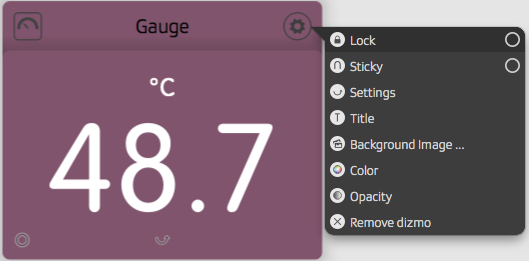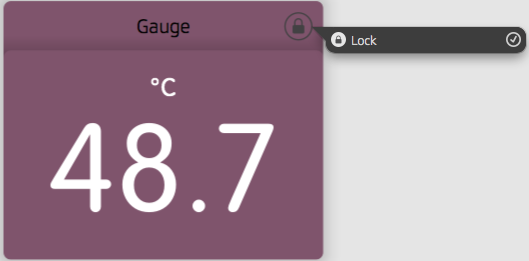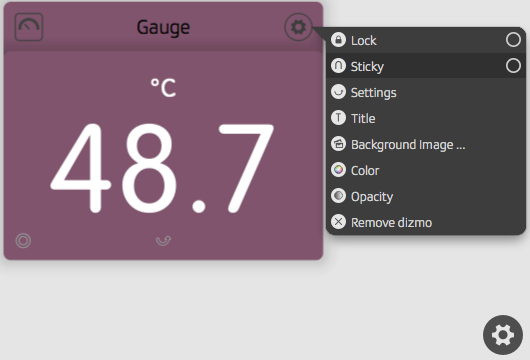# Lock a dizmo and make it sticky

As described in the chapter Hierarchies, the user can arrange dizmos in hierarchies and in a spatial order. In order not to lose this particular spatial order, the user can make a dizmo sticky in the dizmo settings. If the user wishes to not accidentally modify the dizmo, they can lock the dizmo in the dizmo settings.

## Lock a dizmoLock a dizmo

When a dizmo is locked, it can not be dragged around and it can not be modified by the user. As illustrated in the picture below, the settings only show that the dizmo is locked. Only when the user unlocks the dizmo (that is, click on "Lock" the only available item in settings), can they access the other items in settings and modify the dizmo again.Locked dizmo

You can set your dizmo to locked:

``dizmo.setAttribute('state/locked', true);``

## Make a dizmo stickySticky

Once a dizmo is sticky, it always keeps it position relative to the four corners of dizmoViewer. Even if the user zooms out of dizmoViewer, drags it around or resizes it, the sticky dizmo always remains in the same spot.

You can provision that your dizmo is set to sticky flag, so that it always remains on the spot by using

``dizmo.setAttribute('state/sticky', true);``

Remember that you have to make provisions for the geometry, when a dizmo is sticky:

``````dizmo.setAttribute("stickyGeometry/angle", value);
dizmo.setAttribute("stickyGeometry/x", value);
dizmo.setAttribute("stickyGeometry/y", value);
dizmo.setAttribute("stickyGeometry/zoom", value);``````

## Sticky geometry and UI controls

The UI controls location can be addressed in sticky geometry using the pixel information from the following sub-sections (pseudocode):

### Cogwheel button

• x = width - ((zoom=1.0)*(center-x=19px) + (margin-right=11px))
• y = height - ((zoom=1.0)*(center-y=19px) + (margin-bottom=11px))
• radius = (zoom=1.0)*(radius=19px)

### Keyboard button

• x = width/2.0 + ((zoom=1.0)*(center-x=66.5) + (margin-right=0))
• y = height - ((zoom=1.0)*(center-y=19px) + (margin-bottom=11px))
• radius = (zoom=1.0)*(radius=19px)

### Creator button

• x = width/2.0 - ((zoom=1.0)*(center-x=66.5) + (margin-right=0))
• y = height - ((zoom=1.0)*(center-y=19px) + (margin-bottom=11px))
• radius = (zoom=1.0)*(radius=19px)

### Width, height and zoom

The value `width` is by default the width of the dizmoViewer window, while the value of `height` is the height of it. The value of `zoom=1.0` is default, but can be made adaptive using:

``````var scale = parseFloat(
ViewerCore.getProperty('/viewer/attributes/settings/uiscaling'));
var dpi = parseFloat(
ViewerCore.getProperty('/viewer/attributes/system/logicaldpi'));

var zoom = scale * dpi / 96.0;``````